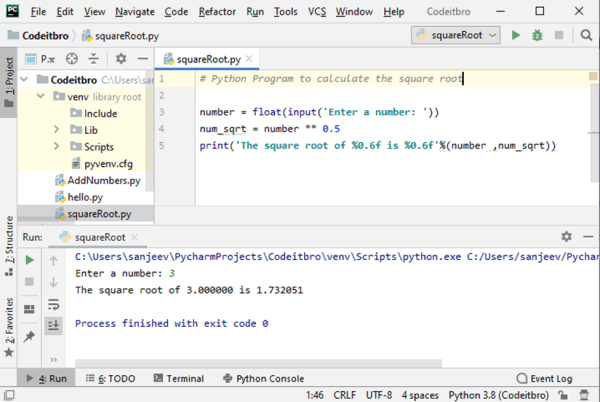# Python 3 Program to Find the Square Root of A Number

3638

In this tutorial, you will learn a simple Python 3 program to find the square root of a number. To understand this program, you should have the knowledge of basic Python programming concepts such as variables and operators.

## Python 3 Program to Find the Square Root of A Number

The ** operator in Python is used to raise the number on the left to the power of the exponent of the right. That is, in the expression 5 ** 3, 5 is being raised to the 3rd power. In mathematics, we often see this expression rendered as 5³, and what is really going on is 5 is being multiplied by itself 3 times. Therefore, to find the square of a number in Python 3, we will raise a number to the power of 0.5.

### Code

```# Python Program to calculate the square root

# Note: change this value for a different result
#num = 8

# uncomment to take the input from the user
num = float(input('Enter a number: '))
num_sqrt = num ** 0.5
print('The square root of %0.3f is %0.3f'%(num ,num_sqrt))
```

### Output

Enter a number: 3

The square root of 3.00000 is 1.732051

Process finished with exit code 0.